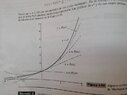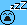# Why is Big-O about how rapidly the Taylor graph approaches that of f(x)?

• B
• mcastillo356

#### mcastillo356

Gold Member
TL;DR Summary
Don't understand Big-O Notation mathematical meaning
Hi, PF
For example, ##\sin{x}=O(x)## as ##x\rightarrow{0}## because ##|\sin{x}|\leq{|x|}## near 0. This fits textbook definition; easy, I think.
But, Taylor's Theorem says that if ##f^{(n+1)}(t)## exists on an interval containing ##a## and ##x##, and if ##P_{n}## is the ##n##th-order Taylor polynomial for ##f## at ##a##, then, as ##x\rightarrow{a}##,
$$f(x)=P_{n}(x)+O\Big({(x-a)^{n+1}}\Big)$$
and "this is a statement about how rapidly the graph of the Taylor polynomial ##P_{n}(x)## aproaches that of ##f(x)## as ##x\rightarrow{a}##; the vertical distance between the graphs decreases as fast as ##|x-a|^{n+1}##.
Italic words is what I don't understand
My attempt is somehow to restrict it to one example: MacLaurin polynomial for ##e^x##:
$$e^x=1+x+\dfrac{x^2}{2!}+\dfrac{x^3}{3!}+\ldots{+\dfrac{x^n}{n!}+O(x^{n+1})}$$
Why the graph decreases as fast as ##|x|^{n+1}##?
Thanks! Hope LaTeX is right and message is understandable (post without preview, sorry).Sorry, I just got it: I must only substitute ##x## and ##n## ... Well, let's see, suppose ...If I look at the graph, and I want to know the value of ##e^{1}## for ##P_{4}##, I get the sequence, I mean, the Maclaurin formula with errors in Big-O form...Let me think it over, sleep on it.Hi PF, working on it. I think must make an effort I haven't made still.
Greetings

This follows from any of the explicit expressions for the remainder. For example, if $$f(x) = f(a) + (x - a)f'(a) + \dots + \frac{(x - a)^n}{n!}f^{(n)}(a) + R_n(x)$$ then by applying the mean value theorem to $$F(t) = f(t) + (x - t)f'(t) + \dots + \frac{(x - t)^n}{n!} f^{(n)}(t)$$ it follows that there exists $\xi$ between $a$ and $x$ such that $$\begin{split} R_n(x) &= F(x) - F(a) \\ &= (x- a)F'(\xi) \\ &= (x - a) \frac{(x - \xi)^{n}}{n!}f^{(n+1)}(\xi).\end{split}$$

••vanhees71, WWGD and mcastillo356
Hi, PF, @pasmith, not familiar to ##\xi##: been trying, though. There goes my attempt. First I quote the texbook, and then my point of view:

"Big-O Notation

We write ##f(x)=O(u(x))## as ##x\rightarrow{a}## provided that
##|f(x)|\leq{k|u(x)|}##
holds for some constant ##k## on some open interval containing ##x=a##.
Similarly, ##f(x)=g(x)+O(u(x))## as ##x\rightarrow{a}## if ##f(x)-g(x)=O(u(x))## as ##x\rightarrow{a}##, that is, if ##|f(x)-g(x)|\leq{k|u(x)|}## near ##a##.
For example, ##\sin{\;x}=O(x)## as ##x\rightarrow{0}## because ##|\sin{\;x}|\leq{|x|}## near 0
The following properties of big-O notation follow from the definition:
(i) If ##f(x)=O(u(x))## as ##x\rightarrow{a}##, then ##Cf(x)=O(u(x))## as ##x\rightarrow{a}## for any value of the constant ##C##.
(ii) If ##f(x)=O(u(x))## as ##x\rightarrow{a}## and ##g(x)=O(u(x))## as ##x\rightarrow{a}##, then ##f(x)\pm{g(x)}=O(u(x))## as ##x\rightarrow{a}##.
(iii) If ##f(x)=O((x-a)^{k}(u(x))## as ##x\rightarrow{a}##, then ##f(x)/(x-a)^{k}=O(u(x))## as ##x\rightarrow{a}## for any constant ##k##
Taylor's Theorem says that if ##f^{(n+1)}(t)## exists on an interval containing ##a## and ##x##, and if ##P_{n}## is the ##n##th-order Taylor polynomial for ##f## at ##a##, then, as ##x\rightarrow{a}##,

##f(x)=P_{n}(x)+O((x-a)^{n+1})##

This is a statement about how rapidly the graph of the Taylor polynomial ##P_{n}(x)## approaches that of ##f(x)## as ##x\rightarrow{a}##; the vertical distance between the graphs decreases as fast as ##|x-a|^{n+1}##."

This precalculus quote is been useful to me. Eventually, in a simpler way, I think I've come to understand the quote, and outline the answer to my question on the first post of the thread: why the distance between graphs getting close to ##f(x)## decreases as fast as ##|x-a|^{n+1}##?:

##f(x)=P_{n}(x)+O((x-a)^{n+1})##
##\Rightarrow{|f(x)-P_{n}(x)|\leq{k|(x-a)^{n+1}|}}##

where ##k=\dfrac{E_{n}(x)}{(x-a)^{n+1}}=\dfrac{f^{(n+1)}(s)}{(n+1)!}##, for some ##s## between ##a## and ##x##

Edited, reason: weird LaTeX used. Check it, PF, please.

Last edited:
•WWGD and pbuk
weird LaTeX used.
"You can't use 'macro parameter character #' in math mode" means you used a single # character to end some inline maths instead of two. It will be around where your post starts to look wierd.

•mcastillo356
Hi, PF, @pasmith: I've been studying, trying to understand your post, and I don´t know if I am right: @pasmith , your post is about Taylor's Theorem with Cauchy's Remainder?
https://tartarus.org/gareth/maths/Analysis_1/Taylors_Theorem.pdf
If so, I would like to ask: why is the key to understand this thread's question? I think that I am bitting off more than I can chew, I mean I'm full of doubts.
My decission is to look for a math teacher, here where I live at. It is the wiser option.
But the main question is: Is it Taylor's Theorem with Cauchy's Remainder?
Thanks. Excuse my poor EnglishHi, PF
This follows from any of the explicit expressions for the remainder. For example, if $$f(x) = f(a) + (x - a)f'(a) + \dots + \frac{(x - a)^n}{n!}f^{(n)}(a) + R_n(x)$$ then by applying the mean value theorem to $$F(t) = f(t) + (x - t)f'(t) + \dots + \frac{(x - t)^n}{n!} f^{(n)}(t)$$ it follows that there exists $\xi$ between $a$ and $x$ such that $$\begin{split} R_n(x) &= F(x) - F(a) \\ &= (x- a)F'(\xi) \\ &= (x - a) \frac{(x - \xi)^{n}}{n!}f^{(n+1)}(\xi).\end{split}$$
Nice quote indeed. I've tried to understand it; prove it, actually. There it goes:

1st- Apply the Mean Value Theorem to $$F(t) = f(t) + (x - t)f'(t) + \dots + \frac{(x - t)^n}{n!} f^{(n)}(t)$$ in the interval ##[a,x]##, or ##[x,a]## (depending on ##a<x## or ##x<a##); chosen ##a<x##, this is, $$F(x)-F(a)=F'(\xi)(x-a)$$
Tasks: ##F(x)-F(a)## is in fact the difference between the function and its Taylor ##n##th-order polynomial?; next, compute ##F'(\xi)##

(i) ##F(a)## is, indeed, ##P_{n}(x)##, this is, the ##n##-th order Taylor polynomial for ##F(x)## at ##x=a##;
(ii) Compute ##F'(\xi)##

Proof by induction

1-for ##n=0##: ##F'(t)=f'(t)=\dfrac{(x-t)^n}{n!}f^{(n+1)}(t)##.
2-for ##n=1##: ##F'(t)=f'(t)+(x-t)f''(t)-f'(t)=\dfrac{(x-t)^n}{n!}f^{(n+1)}(t)##

Inductive step

Suppose proven for ##n##, and prove it for ##n+1##:

We got

##F(t)=\displaystyle\sum_{k=0}^n\dfrac{(x-t)^k}{k!}f^{(k)}(t)##

##F(t)=\displaystyle\sum_{k=1}^{n+1}{\dfrac{(x-t)^{k}}{k!}f^{(k)}}(t)=\underbrace{\displaystyle\sum_{k=1}^{n}{\dfrac{(x-t)^{k}}{k!}f^{(k)}}(t)}_{*}+\dfrac{(x-t)^{n+1}}{(n+1)!}f^{(n+1)}(t) ##

It is possible to apply the induction hypotesis on ##(*)## when differentiating. Renders

##F'(t)=\underbrace{\dfrac{(x-t)^n}{n!}f^{(n+1)}(t)}_*-\dfrac{(x-t)^n}{n!}f^{(n+1)}(t)+\dfrac{(x-t)^{n+1}}{(n+1)!}f^{(n+2)}(t)=\dfrac{(x-t)^{n+1}}{(n+1)!}f^{(n+2)}(t)##

Proven, See you later. Still got some doubts. Take this post like some kind of rough draft.

Greetings. Please check LaTeX, post without preview

Peace, love.

Marcos

Hi, PF, hope not to be cumbersome, but I've come to the conclusion that this thread lacks of completeness...Well, this word might not be appropiate; I mean coherence, consistency:

Must be provided a proof for the mentioned three properties of the Big O notation;
There is a Taylor's Theorem quote that leads to Theorem 13, mentioned in this thread, but not justified; I mean explained in detail.

I'll give a trie in the next posts.

Peace and Love!

"Big-O Notation

We write ##f(x)=O(u(x))## as ##x\rightarrow{a}## provided that
##|f(x)|\leq{k|u(x)|}##
holds for some constant ##k## on some open interval containing ##x=a##.
Similarly, ##f(x)=g(x)+O(u(x))## as ##x\rightarrow{a}## if ##f(x)-g(x)=O(u(x))## as ##x\rightarrow{a}##, that is, if ##|f(x)-g(x)|\leq{k|u(x)|}## near ##a##.
For example, ##\sin{\;x}=O(x)## as ##x\rightarrow{0}## because ##|\sin{\;x}|\leq{|x|}## near 0
The following properties of big-O notation follow from the definition:
(i) If ##f(x)=O(u(x))## as ##x\rightarrow{a}##, then ##Cf(x)=O(u(x))## as ##x\rightarrow{a}## for any value of the constant ##C##.

(i)##Cf(x)=O(u(x))\Rightarrow{|Cf(x)|\leq{k|u(x)|}}\Rightarrow{|f(x)|\leq{k|u(x)|}\leq{\dfrac{k}{C}|u(x)|}}\Rightarrow{\dfrac{k}{C}>0}##

(ii) If ##f(x)=O(u(x))## as ##x\rightarrow{a}## and ##g(x)=O(u(x))## as ##x\rightarrow{a}##, then ##f(x)\pm{g(x)}=O(u(x))## as ##x\rightarrow{a}##.
(ii)
Got ##|f(x)|\leq{k|u(x)|}## and ##|g(x)|\leq{k'|u(x)|}##. Thus, ##|f(x)\pm{g(x)}|\leq{|f(x)|+|g(x)|}\leq{(k+k')}|u(x)|##

(iii) If ##f(x)=O((x-a)^{k}(u(x))## as ##x\rightarrow{a}##, then ##f(x)/(x-a)^{k}=O(u(x))## as ##x\rightarrow{a}## for any constant ##k##

##f(x)=O((x-a)^{k}(u(x))\Leftrightarrow{|f(x)|\leq{k|(x-a)^{k}u(x)|}}\Leftrightarrow{f(x)/(x-a)^{k}}=O(u(x))##

Still, @mcastillo356 , notice Sinx is somewhat of a special case, as ## |sinx|\leq 1##

•mcastillo356
Still, @mcastillo356 , notice Sinx is somewhat of a special case, as ## |sinx|\leq 1##
@WWGD, very interesting your mention, but what difference does it make? I mean, trigonometric inverse functions are not my aim. In fact ##\sin{\;x}## is just an example. The reason for it to appear is that helps introducing Big ##O## inmediately, just as ##\mbox{Sin y}##. My aim is, at this first step, explore Big ##O##; but the domain of the functions will remain at the abscissas.

Last edited:
Taylor's Theorem says that if ##f^{(n+1)}(t)## exists on an interval containing ##a## and ##x##, and if ##P_{n}## is the ##n##th-order Taylor polynomial for ##f## at ##a##, then, as ##x\rightarrow{a}##,

##f(x)=P_{n}(x)+O((x-a)^{n+1})##

This is a statement about how rapidly the graph of the Taylor polynomial ##P_{n}(x)## approaches that of ##f(x)## as ##x\rightarrow{a}##; the vertical distance between the graphs decreases as fast as ##|x-a|^{n+1}##.
I don't understand this quote. My attempt:

##f(x)-P_{n}(x)=O\Big(|x-a|^{n+1}\Big)##. On the right side there is the error term, the rapidness Taylor's polynomial approaches to ##f(x)##, and third:
$$|f(x)-P_{n}(x)|\leq{k|x-a|^{n+1}}$$
That means it exists a constant ##k## such that the distance between the function and the Taylor polynomial is bounded by a multiple of the function ##|x-a|^{n+1}##. I can't help watching these three approaches as something not compatible, although I know it must be.

Last edited:
Hi, PF

$$f(x)-P_{n}(x)=O\Big(|x-a|^{n+1}\Big)\Rightarrow{|f(x)-P_{n}(x)|\leq{k|x-a|^{n+1}}}$$

If there exists a constant ##k## bounding ##|f(x)-P_{n}(x)|## by ##|x-a|^{n+1}##, we've got an order of approximation; this means an expression for how accurate the Taylor polynomial comes near to a function. Don't you think talking about accuracy is more precise than rapidlity?

Don't you think talking about accuracy is more precise than rapidlity?
No, I think there must be some linguistic confusion. You are looking at two sides of the same coin and thinking it must be a different coin.

Because ## f(x)=P_{n}(x)+O((x-a)^{n+1}) \iff |f(x)-P_{n}(x)|\leq{k|x-a|^{n+1}} ## we can talk about accuracy and rapidity interchangeably.

••FactChecker and mcastillo356
It is a three sides coin, error, rapidity or accuracy, and order of approximation

It is a three sides coin, error, rapidity or accuracy, and order of approximation
You should not come away with the impression that the definition is sloppy. The casual descriptions here are a little deceptive. There are some formal definitions of the Big O notation that are quite specific. The details may differ among mathematicians, but each one is precise.

••mcastillo356 and pbuk
Hi, PF, Big O notation seem it is understood, I'm going to publish the last obstacle, reef or rock where I stumble; the Theorem 13 at Calculus-A complete course 7th edition, R. Adams and C. Essex, Section 4.10, Taylor polynomials, It happens to be another thread I will untitle "Understanding T. 13, from the textbook Calculus, R.Adams and C. Essex, 7th edition, 4.10"

Love